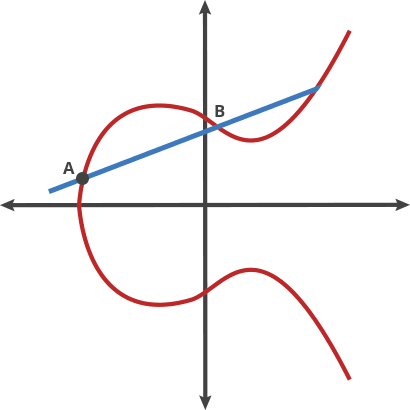## Generate Private Key1. Private key is 256 random bits.
80
01
2. Prepend version number.
3. Append compression flag.
4. Append checksum. Checksum is the first 4 bytes of double sha256 hash of whatever is being checkedsum'ed.5. Base58 encoded data is easier to read and manage. The "double-arrows" indicate this is reversible.

## Generate Public Key

k
= *6. Multiply the private key by the elliptic curve generator point to get the public key. The public key is a point on the elliptic curve and has x and y coordinates.x =
y =
N/
A
7. Use parity of y coordinate and full x coordinate to represent the public key.N/A
8. Hash public key twice. This obfuscates the public key and shortens it.
00
9. Prepend version (version number is different than in step 2)
10. Append checksum (same method as step 4)N/A
11. After another base58 encoding, we have our public address :)